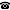# 2019 seminar talk: The Golomb space is topologically rigid

Talk held by Taras Banakh (Ivan Franko National University of Lviv, Ukraine) at the KGRC seminar on 2019-12-19.

### Abstract

The Golomb space is the space $\mathcal N$ of natural numbers endowed with the topology generated by the base consisting of arithmetic progressions $a+b\mathbb N$ with coprime parameters $a$ and $b$. The Golomb space is one of the simplest examples of a countable connected Hausdorff space. In this space Topology and Arithmetic are tightly intertwined. We survey topological properties of the Golomb space and prove that this space is topologically rigid, i.e., has trivial homeomorphism group. This resolves a problem posed on Mathoverflow in 2017. Philosophically, the topological rigidity of the Golomb space can be interpreted as the possibility to encode in Topology all Arithmetics (which encodes all Mathematics by the Godel arithmetization).

Kurt Gödel Research Center for Mathematical Logic. Währinger Straße 25, 1090 Wien, Austria.+43-1-4277-50501. Last updated: 2010-12-16, 04:37.